Скачать презентацию Thinking the Impossible Modern Cryptography Jeremy R Johnson

12c7ea03f93fd4bfc526d404128c5a59.ppt

• Количество слайдов: 30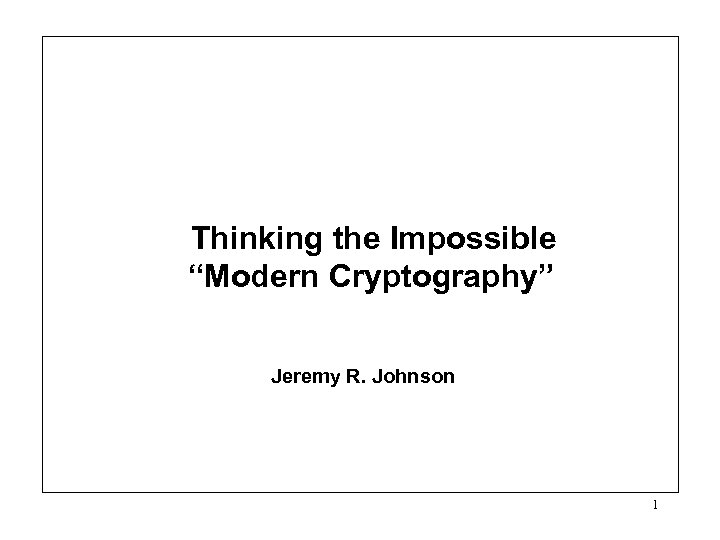Thinking the Impossible “Modern Cryptography” Jeremy R. Johnson 1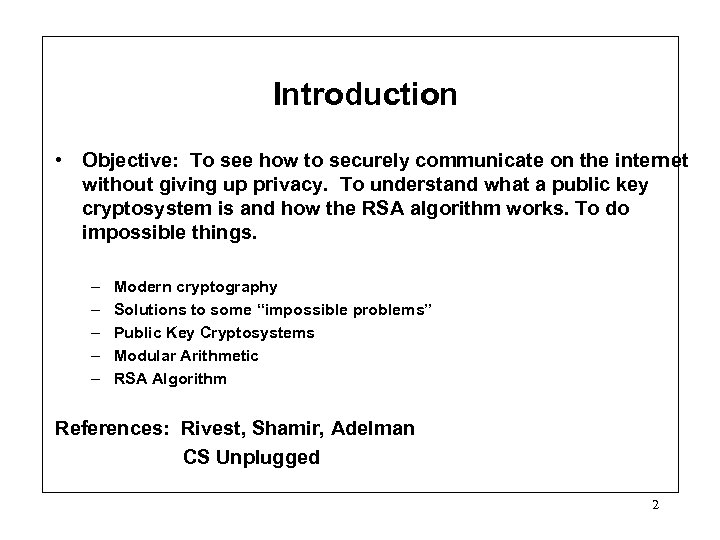Introduction • Objective: To see how to securely communicate on the internet without giving up privacy. To understand what a public key cryptosystem is and how the RSA algorithm works. To do impossible things. – – – Modern cryptography Solutions to some “impossible problems” Public Key Cryptosystems Modular Arithmetic RSA Algorithm References: Rivest, Shamir, Adelman CS Unplugged 2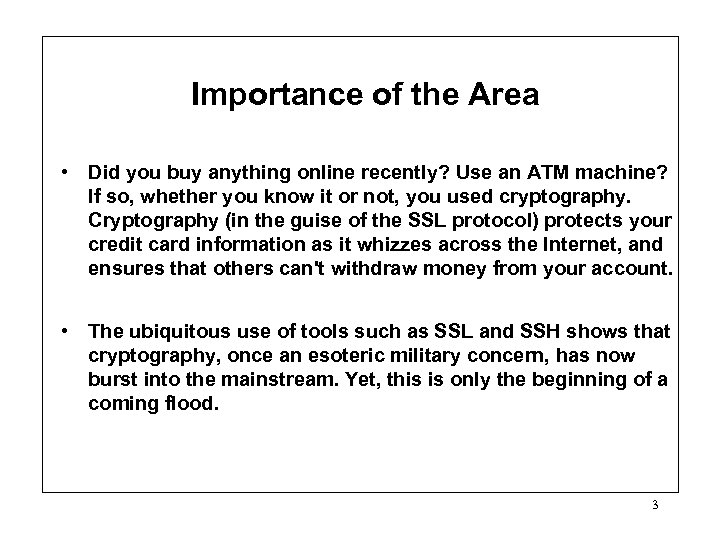Importance of the Area • Did you buy anything online recently? Use an ATM machine? If so, whether you know it or not, you used cryptography. Cryptography (in the guise of the SSL protocol) protects your credit card information as it whizzes across the Internet, and ensures that others can't withdraw money from your account. • The ubiquitous use of tools such as SSL and SSH shows that cryptography, once an esoteric military concern, has now burst into the mainstream. Yet, this is only the beginning of a coming flood. 3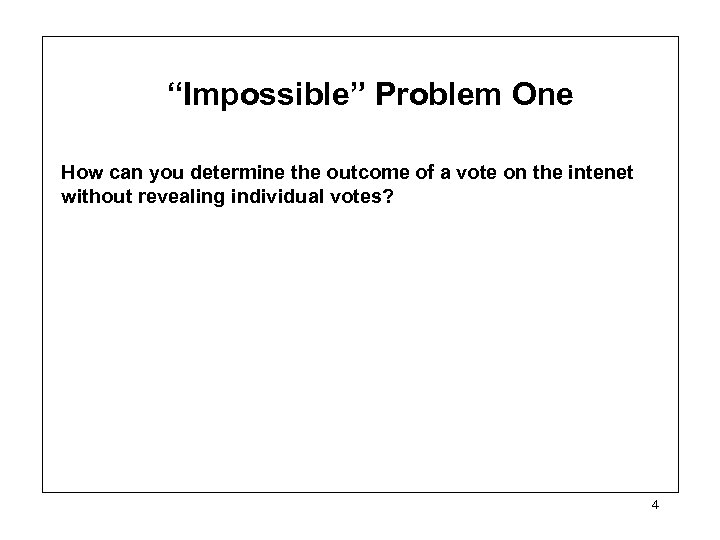“Impossible” Problem One How can you determine the outcome of a vote on the intenet without revealing individual votes? 4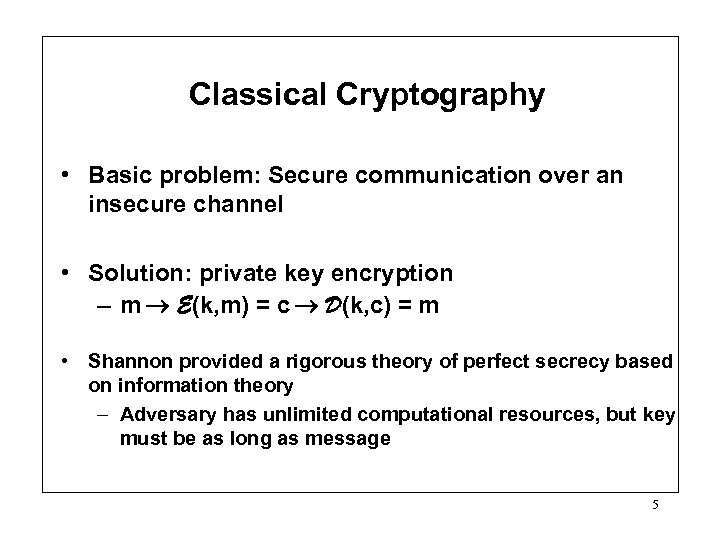Classical Cryptography • Basic problem: Secure communication over an insecure channel • Solution: private key encryption – m E(k, m) = c D(k, c) = m • Shannon provided a rigorous theory of perfect secrecy based on information theory – Adversary has unlimited computational resources, but key must be as long as message 5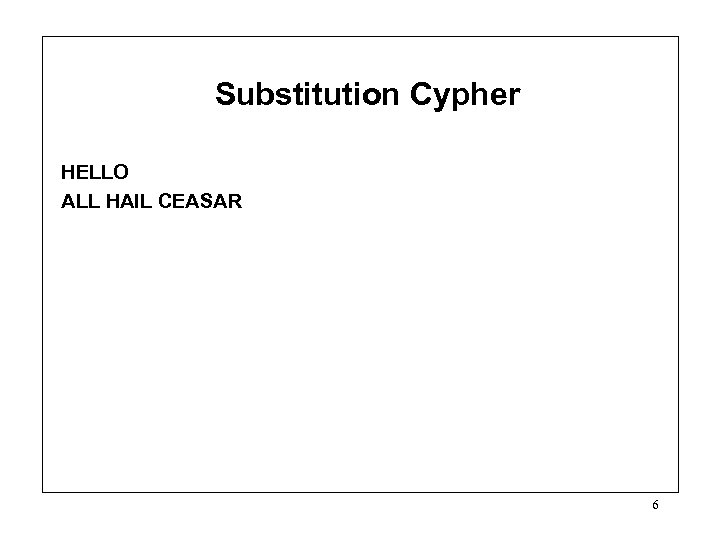Substitution Cypher HELLO ALL HAIL CEASAR 6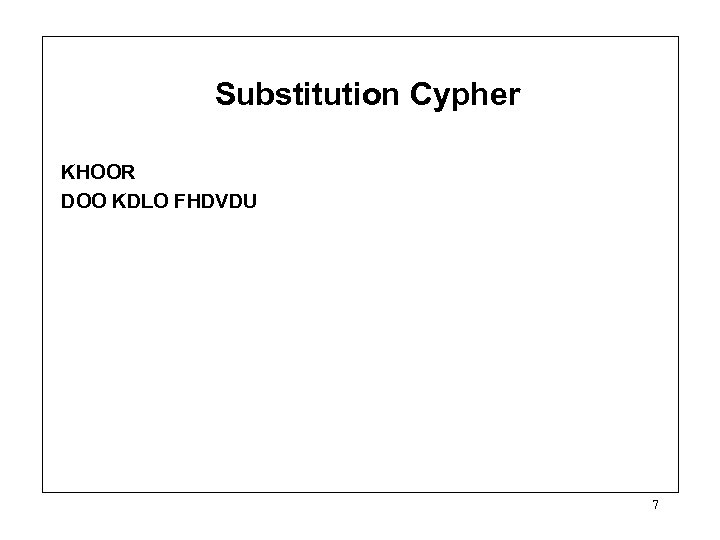Substitution Cypher KHOOR DOO KDLO FHDVDU 7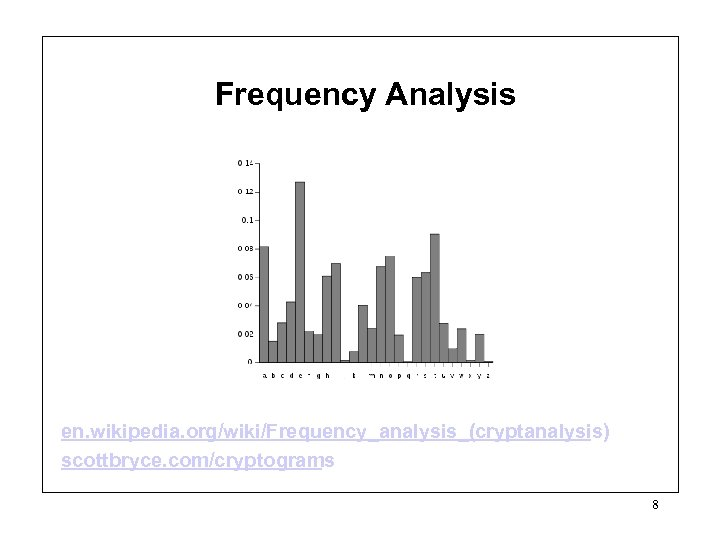Frequency Analysis en. wikipedia. org/wiki/Frequency_analysis_(cryptanalysis) scottbryce. com/cryptograms 8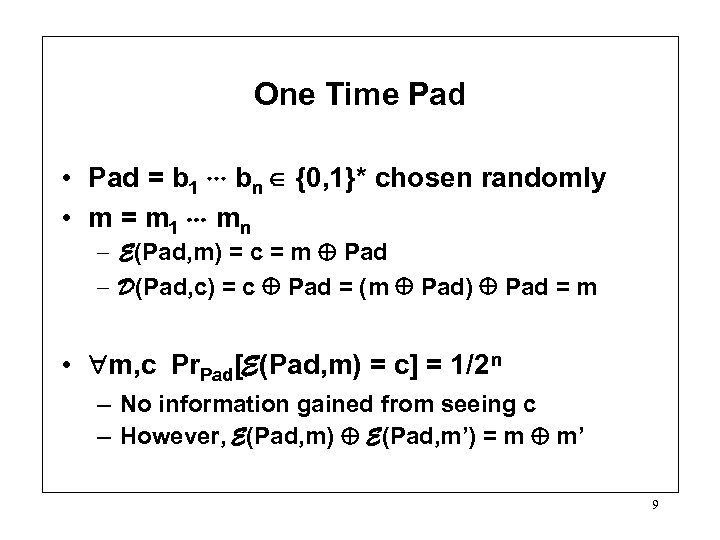One Time Pad • Pad = b 1 bn {0, 1}* chosen randomly • m = m 1 mn – E(Pad, m) = c = m Pad – D(Pad, c) = c Pad = (m Pad) Pad = m • m, c Pr. Pad[E(Pad, m) = c] = 1/2 n – No information gained from seeing c – However, E(Pad, m) E(Pad, m’) = m m’ 9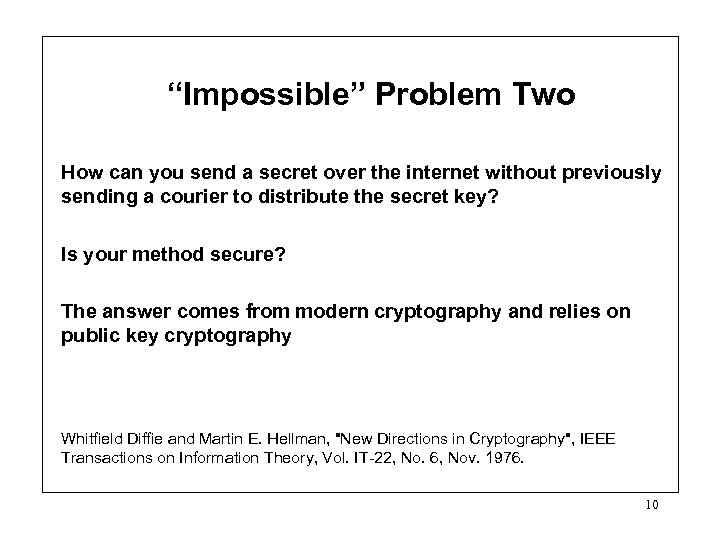“Impossible” Problem Two How can you send a secret over the internet without previously sending a courier to distribute the secret key? Is your method secure? The answer comes from modern cryptography and relies on public key cryptography Whitfield Diffie and Martin E. Hellman, "New Directions in Cryptography", IEEE Transactions on Information Theory, Vol. IT-22, No. 6, Nov. 1976. 10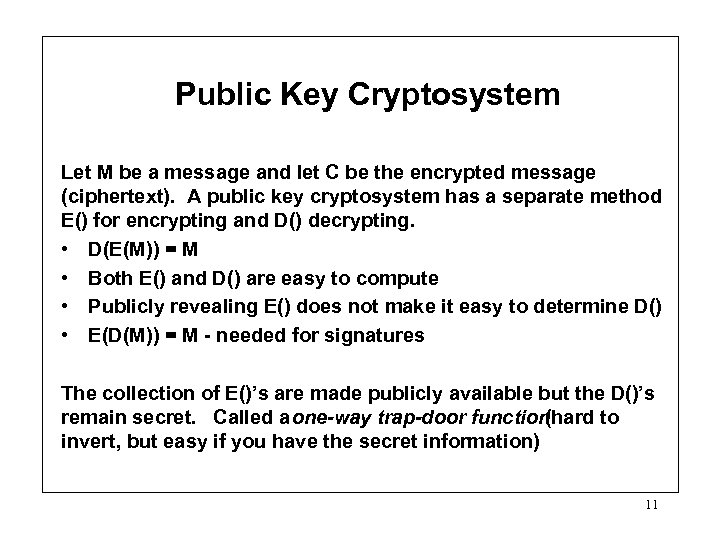Public Key Cryptosystem Let M be a message and let C be the encrypted message (ciphertext). A public key cryptosystem has a separate method E() for encrypting and D() decrypting. • D(E(M)) = M • Both E() and D() are easy to compute • Publicly revealing E() does not make it easy to determine D() • E(D(M)) = M - needed for signatures The collection of E()’s are made publicly available but the D()’s remain secret. Called a one-way trap-door function (hard to invert, but easy if you have the secret information) 11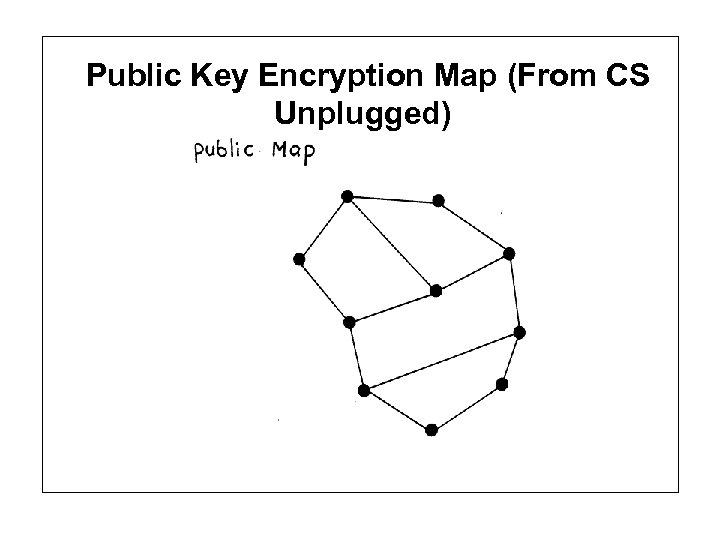Public Key Encryption Map (From CS Unplugged)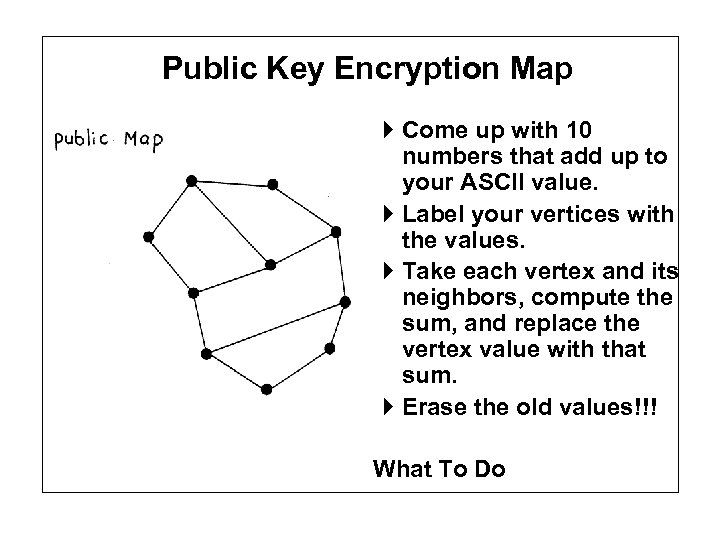Public Key Encryption Map The Map Come up with 10 numbers that add up to your ASCII value. Label your vertices with the values. Take each vertex and its neighbors, compute the sum, and replace the vertex value with that sum. Erase the old values!!! What To Do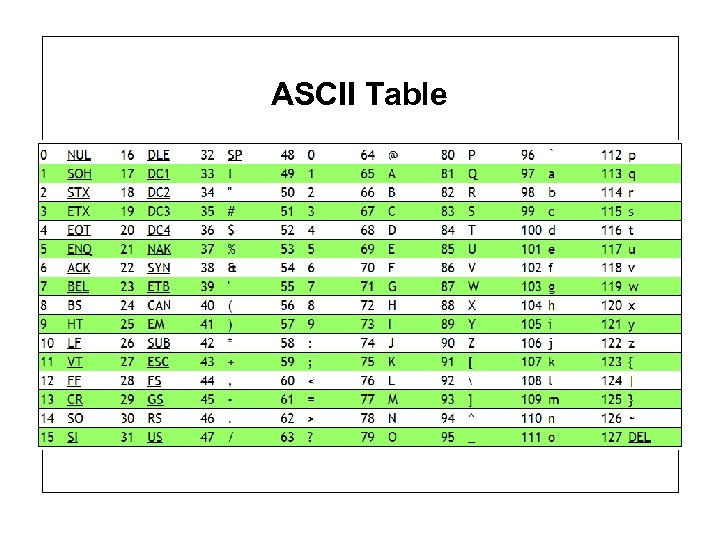ASCII Table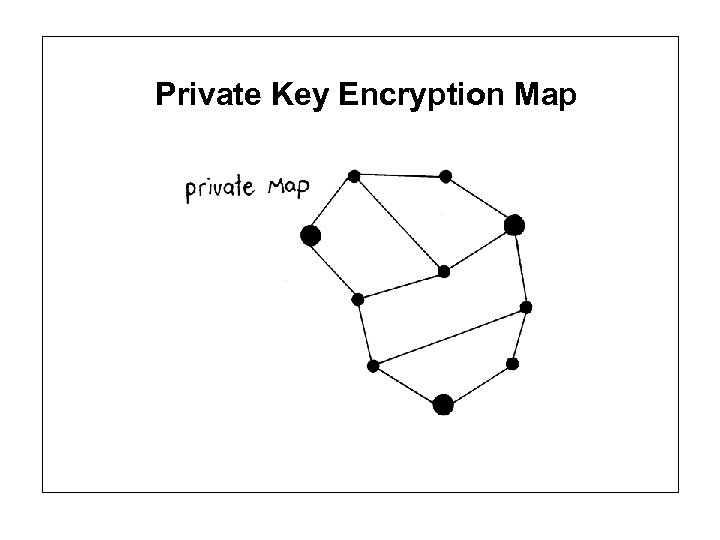Private Key Encryption Map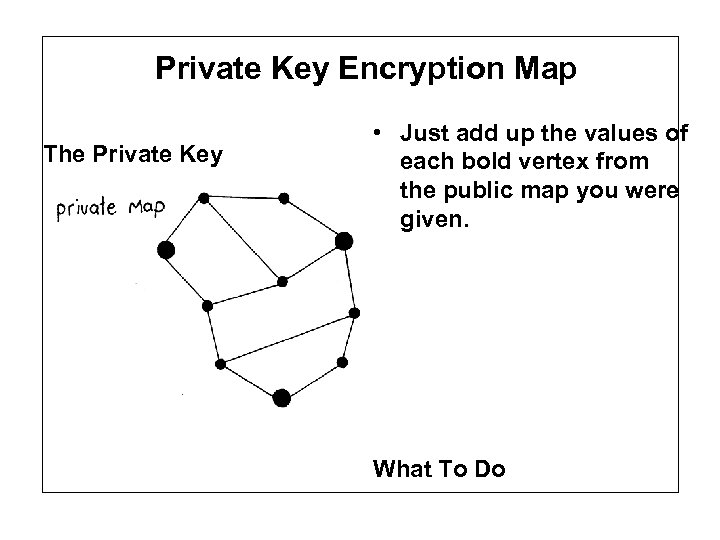Private Key Encryption Map The Private Key • Just add up the values of each bold vertex from the public map you were given. What To Do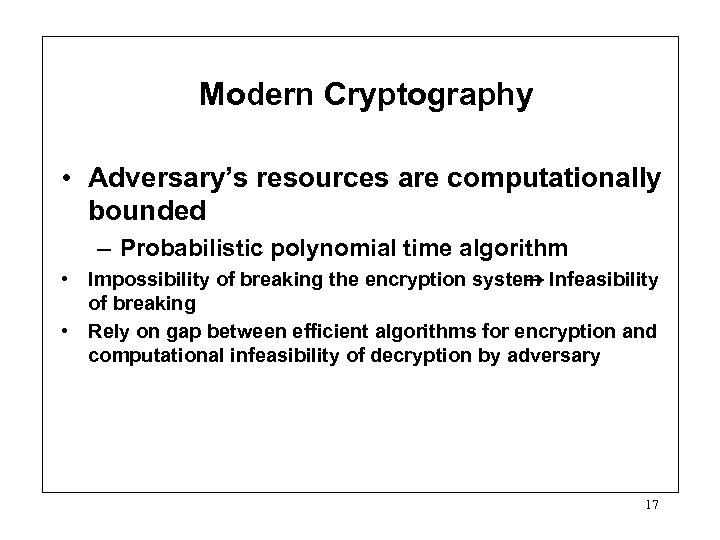Modern Cryptography • Adversary’s resources are computationally bounded – Probabilistic polynomial time algorithm • Impossibility of breaking the encryption system Infeasibility of breaking • Rely on gap between efficient algorithms for encryption and computational infeasibility of decryption by adversary 17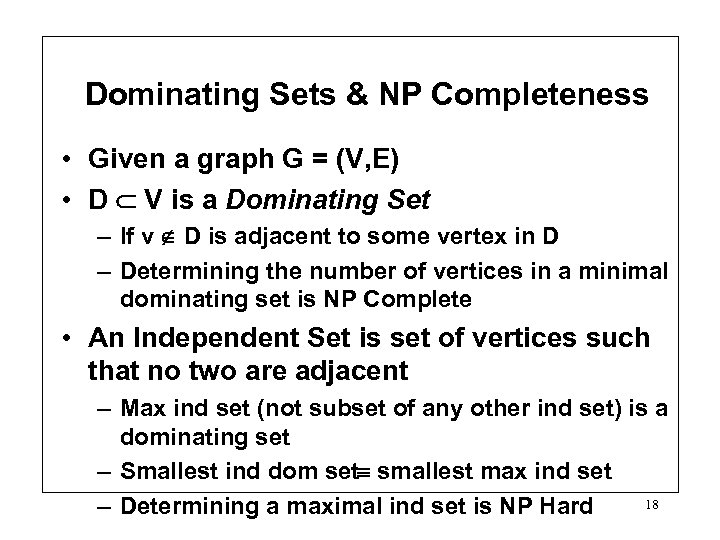Dominating Sets & NP Completeness • Given a graph G = (V, E) • D V is a Dominating Set – If v D is adjacent to some vertex in D – Determining the number of vertices in a minimal dominating set is NP Complete • An Independent Set is set of vertices such that no two are adjacent – Max ind set (not subset of any other ind set) is a dominating set – Smallest ind dom set smallest max ind set 18 – Determining a maximal ind set is NP Hard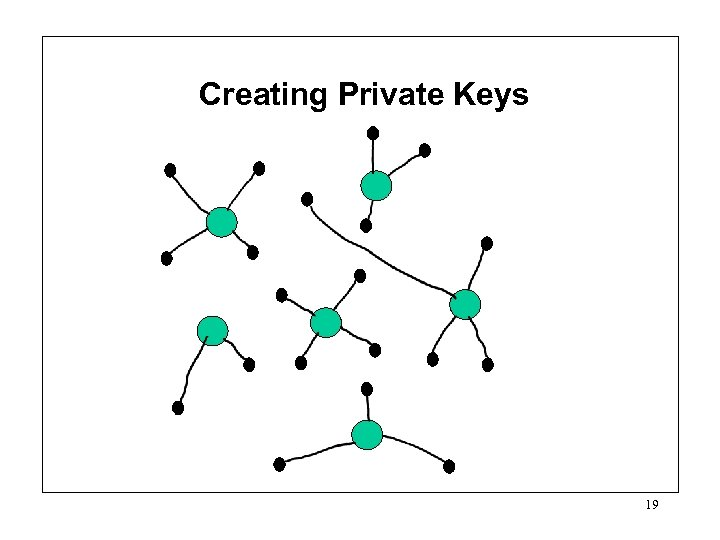Creating Private Keys 19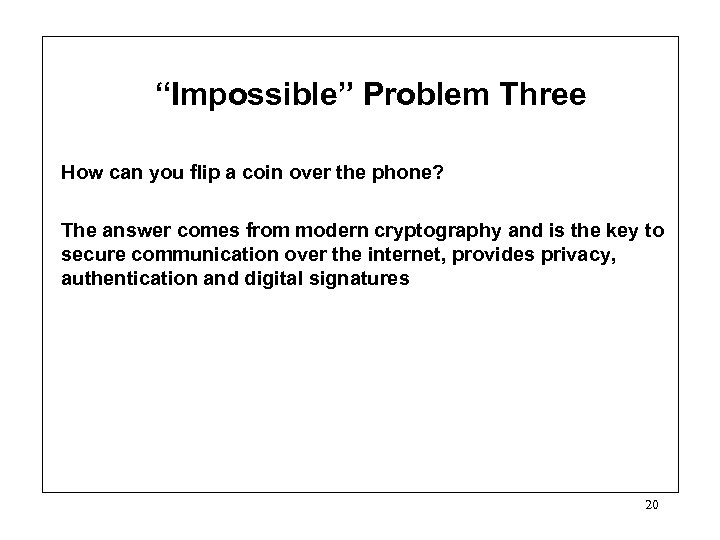“Impossible” Problem Three How can you flip a coin over the phone? The answer comes from modern cryptography and is the key to secure communication over the internet, provides privacy, authentication and digital signatures 20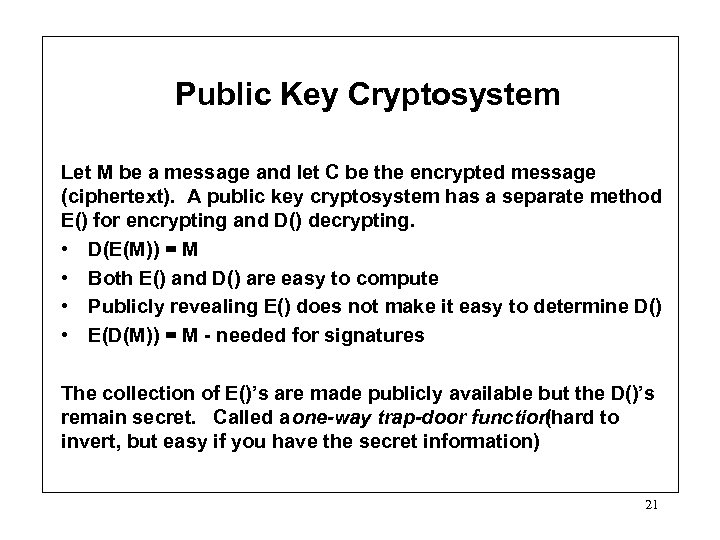Public Key Cryptosystem Let M be a message and let C be the encrypted message (ciphertext). A public key cryptosystem has a separate method E() for encrypting and D() decrypting. • D(E(M)) = M • Both E() and D() are easy to compute • Publicly revealing E() does not make it easy to determine D() • E(D(M)) = M - needed for signatures The collection of E()’s are made publicly available but the D()’s remain secret. Called a one-way trap-door function (hard to invert, but easy if you have the secret information) 21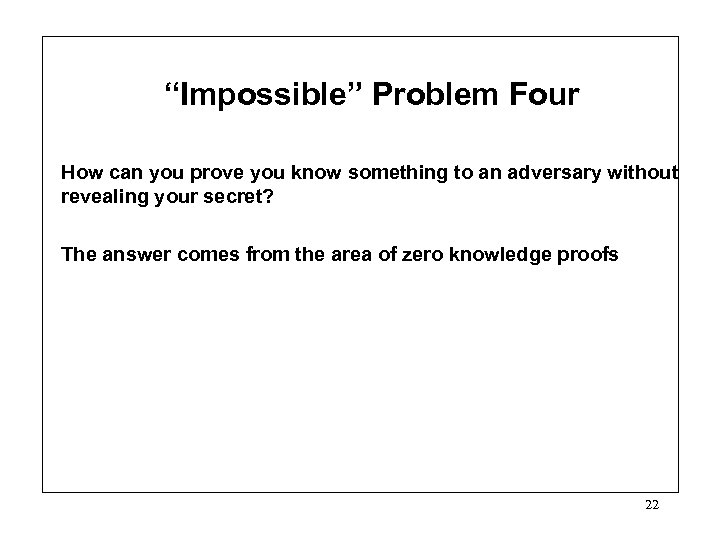“Impossible” Problem Four How can you prove you know something to an adversary without revealing your secret? The answer comes from the area of zero knowledge proofs 22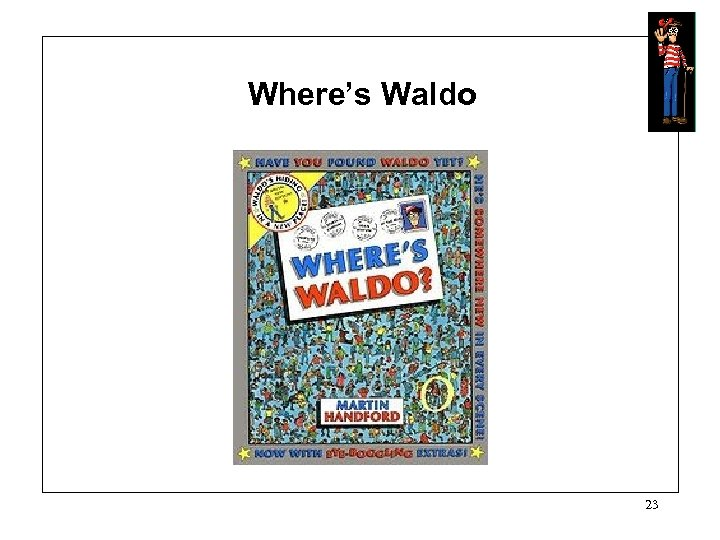Where’s Waldo 23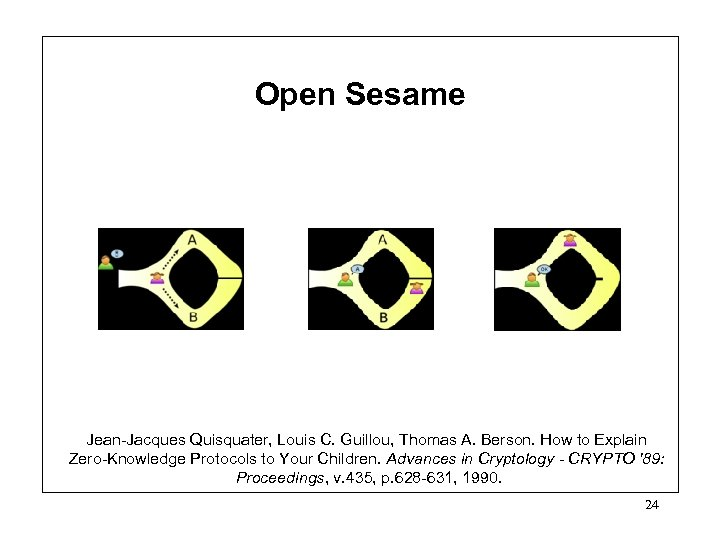Open Sesame Jean-Jacques Quisquater, Louis C. Guillou, Thomas A. Berson. How to Explain Zero-Knowledge Protocols to Your Children. Advances in Cryptology - CRYPTO '89: Proceedings, v. 435, p. 628 -631, 1990. 24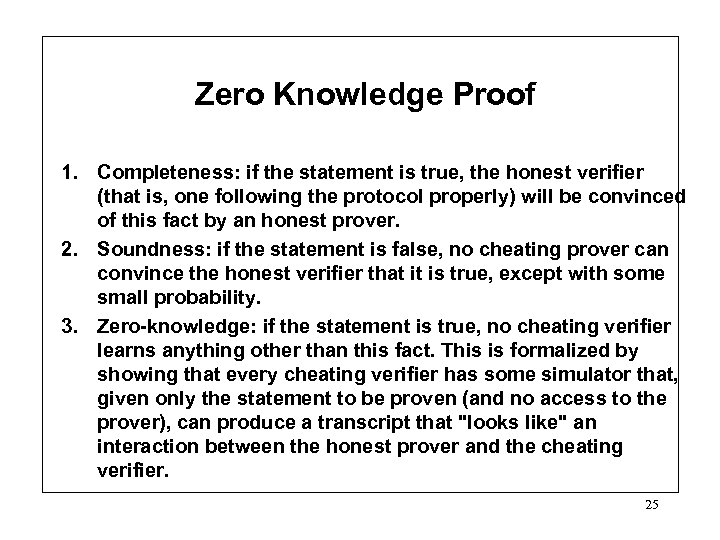Zero Knowledge Proof 1. Completeness: if the statement is true, the honest verifier (that is, one following the protocol properly) will be convinced of this fact by an honest prover. 2. Soundness: if the statement is false, no cheating prover can convince the honest verifier that it is true, except with some small probability. 3. Zero-knowledge: if the statement is true, no cheating verifier learns anything other than this fact. This is formalized by showing that every cheating verifier has some simulator that, given only the statement to be proven (and no access to the prover), can produce a transcript that "looks like" an interaction between the honest prover and the cheating verifier. 25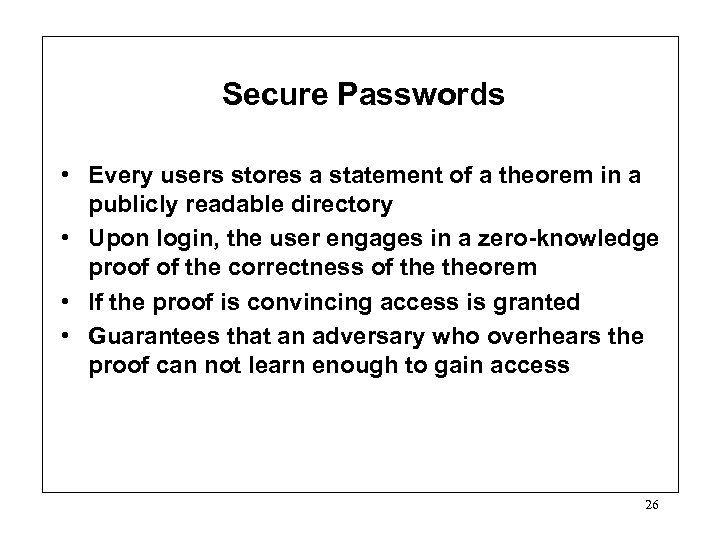Secure Passwords • Every users stores a statement of a theorem in a publicly readable directory • Upon login, the user engages in a zero-knowledge proof of the correctness of theorem • If the proof is convincing access is granted • Guarantees that an adversary who overhears the proof can not learn enough to gain access 26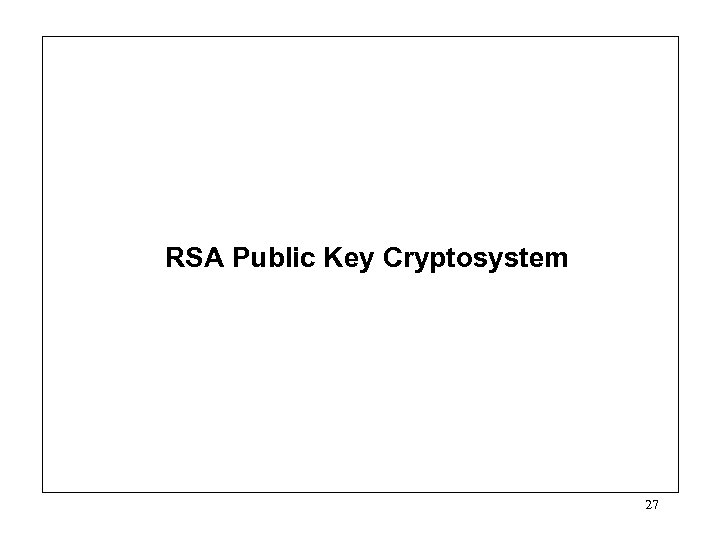RSA Public Key Cryptosystem 27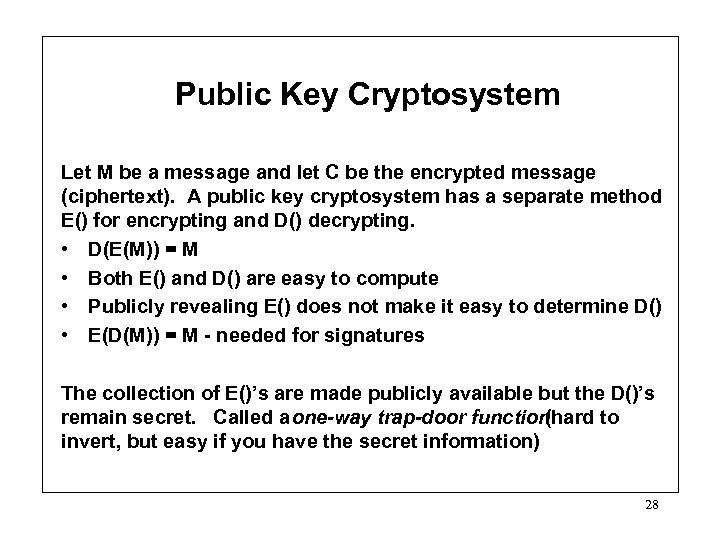Public Key Cryptosystem Let M be a message and let C be the encrypted message (ciphertext). A public key cryptosystem has a separate method E() for encrypting and D() decrypting. • D(E(M)) = M • Both E() and D() are easy to compute • Publicly revealing E() does not make it easy to determine D() • E(D(M)) = M - needed for signatures The collection of E()’s are made publicly available but the D()’s remain secret. Called a one-way trap-door function (hard to invert, but easy if you have the secret information) 28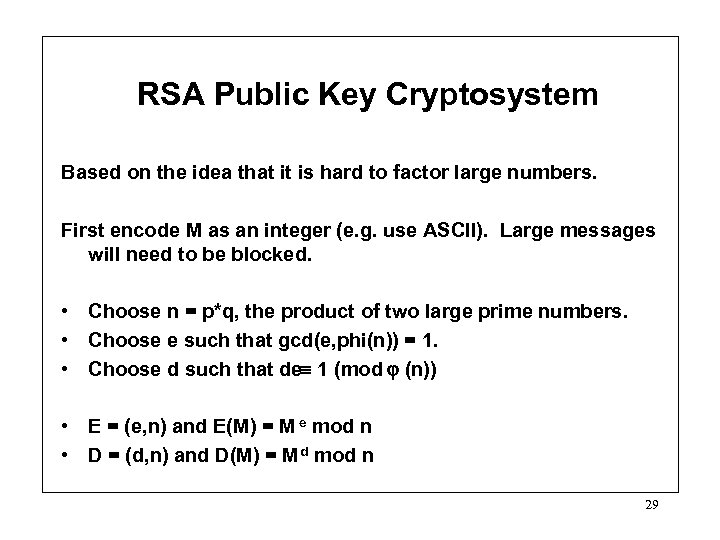RSA Public Key Cryptosystem Based on the idea that it is hard to factor large numbers. First encode M as an integer (e. g. use ASCII). Large messages will need to be blocked. • Choose n = p*q, the product of two large prime numbers. • Choose e such that gcd(e, phi(n)) = 1. • Choose d such that de 1 (mod (n)) • E = (e, n) and E(M) = M e mod n • D = (d, n) and D(M) = M d mod n 29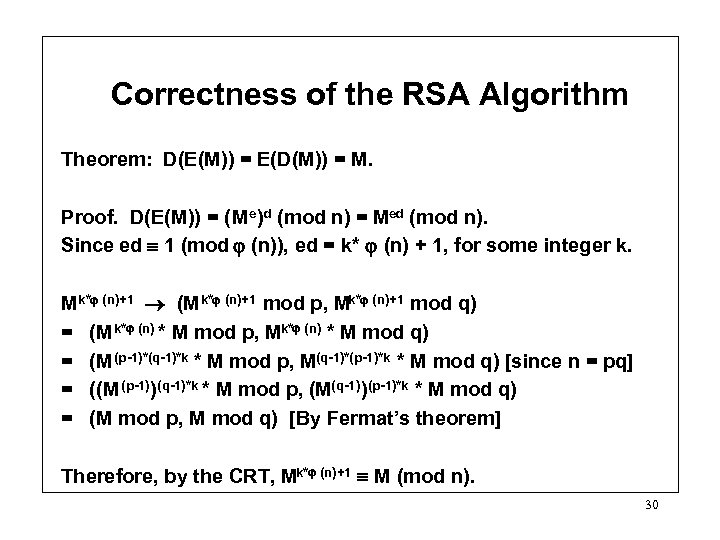Correctness of the RSA Algorithm Theorem: D(E(M)) = E(D(M)) = M. Proof. D(E(M)) = (Me)d (mod n) = Med (mod n). Since ed 1 (mod (n)), ed = k* (n) + 1, for some integer k. Mk* (n)+1 (M k* (n)+1 mod p, Mk* (n)+1 mod q) = (M k* (n) * M mod p, Mk* (n) * M mod q) = (M (p-1)*(q-1)*k * M mod p, M(q-1)*(p-1)*k * M mod q) [since n = pq] = ((M (p-1))(q-1)*k * M mod p, (M(q-1))(p-1)*k * M mod q) = (M mod p, M mod q) [By Fermat’s theorem] Therefore, by the CRT, Mk* (n)+1 M (mod n). 30